# Main Networks

Note

All main networks should inherit from the abstract class hypnettorch.mnets.mnet_interface.MainNetInterface to provide a consistent interface for users.

## Bidirectional Recurrent Neural Network

This module implements a bidirectional recurrent neural networt (BiRNN). To realize recurrent layers, it utilizes class mnets.simple_rnn.SimpleRNN. Hence different kinds of BiRNNs can be realized, such as Elman-type BiRNNs and BiLSTMs. In particular, this class implements the BiRNN in the following manner. Given an input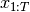, the forward RNN is run to produce hidden states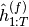and the backward RNN is run to produce states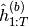.

These hidden states are concatenated to produce the final hidden state which is the output of the recurrent layer(s):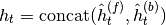.

Those inputs are subsequently processed by an instance of class mnets.mlp.MLP to produce the final network outputs.

class hypnettorch.mnets.bi_rnn.BiRNN(rnn_args={}, mlp_args=None, preprocess_fct=None, no_weights=False, verbose=True)[source]

Implementation of a bidirectional RNN.

Note

The output is non-linear if the last layer is recurrent! Otherwise, logits are returned (cmp. attribute mnets.mnet_interface.MainNetInterface.has_fc_out).

Example

Here is an example instantiation of a BiLSTM with a single bidirectional layer of dimensionality 256, assuming 100 dimensional inputs and 10 dimensional outputs.

net = BiRNN(rnn_args={'n_in': 100, 'rnn_layers': ,
'use_lstm': True, 'fc_layers_pre': [],
'fc_layers': []},
mlp_args={'n_in': 512, 'n_out': 10,
'hidden_layers': []},
no_weights=False)

Parameters:
• rnn_args (dict or list) –

A dictionary of arguments for an instance of class mnets.simple_rnn.SimpleRNN. These arguments will be used to create two instances of this class, one representing the forward RNN and one the backward RNN.

Note, each of these instances may contain multiple layers, even non-recurrent layers. The outputs of such an instance are considered the hidden activationsor, respectively.

To realize multiple bidirectional layers (which in itself can be multi-layer RNNs), one may provide a list of dictionaries. Each entry in such list will be used to generate a single bidirectional layer (i.e., consisting of two instances of class mnets.simple_rnn.SimpleRNN). Note, the input size of each new layer has to be twice the size of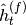from the previous layer.

• mlp_args (dict, optional) – A dictionary of arguments for class mnets.mlp.MLP. The input size of such an MLP should be twice the size of. If None, then the output of the last bidirectional layer is considered the output of the network.

• preprocess_fct (func, optional) –

A function handle can be provided, that will process inputs x passed to the method forward(). An example usecase could be the translation or selection of word embeddings.

The function handle must have the signature: preprocess_fct(x, seq_lengths=None). See the corresponding argument descriptions of method forward().The function is expected to return the preprocessed x.

• no_weights (bool) – See parameter no_weights of class mnets.mlp.MLP.

• verbose (bool) – See parameter verbose of class mnets.mlp.MLP.

Initializes internal Module state, shared by both nn.Module and ScriptModule.

distillation_targets()[source]

Targets to be distilled after training.

See docstring of abstract super method mnets.mnet_interface.MainNetInterface.distillation_targets().

forward(x, weights=None, distilled_params=None, condition=None, seq_lengths=None)[source]

Compute the output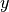of this network given the input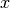.

Note

If constructor argument preprocess_fct was set, then all inputs x are first processed by this function.

Parameters:
• (....) – See docstring of method mnets.mnet_interface.MainNetInterface.forward(). We provide some more specific information below.

• weights (list or dict) – See argument weights of method mnets.mlp.MLP.forward().

• distilled_params – Will only be passed to the underlying instance of class mnets.mlp.MLP

• condition (int or dict, optional) –

If provided, then this argument will be passed as argument ckpt_id to the method utils.context_mod_layer.ContextModLayer.forward().

When providing as dict, see argument condition of method mnets.mlp.MLP.forward() for more details.

• seq_lengths (numpy.ndarray, optional) –

List of sequence lengths. The length of the list has to match the batch size of inputs x. The entries will correspond to the unpadded sequence lengths. If this option is provided, then the bidirectional layers will reverse its input sequences according to the unpadded sequence lengths.

Example

x = [[a,b,0,0], [a,b,c,0]].T. If seq_lengths = [2, 3] if provided, then the reverse sequences [[b,a,0,0], [c,b,a,0]].T are fed into the first bidirectional layer (and similarly for all subsequent bidirectional layers). Otherwise reverse sequences [[0,0,b,a], [0,c,b,a]].T are used.

Caution

If this option is not provided but padded input sequences are used, the output of a bidirectional layer will depent on the padding. I.e., different padding lengths will lead to different results.

Returns:

Where the tuple is containing:

• output (torch.Tensor): The output of the network.

• hidden (list): None - not implemented yet.

Return type:
get_cm_weights()[source]

Get internal maintained weights that are associated with context- modulation.

Returns:

List of weights from mnets.mnet_interface.MainNetInterface.internal_params that are belonging to context-mod layers.

Return type:

(list)

get_non_cm_weights()[source]

Get internal weights that are not associated with context-modulation.

Returns:

List of weights from mnets.mnet_interface.MainNetInterface.internal_params that are not belonging to context-mod layers.

Return type:

(list)

init_hh_weights_orthogonal()[source]

Initialize hidden-to-hidden weights orthogonally.

This method will call method mnets.simple_rnn.SimpleRNN.init_hh_weights_orthogonal() of all internally maintained instances of class mnets.simple_rnn.SimpleRNN.

property num_rec_layers

See attribute mnets.simple_rnn.SimpleRNN.num_rec_layers. Total number of recurrent layer, where each bidirectional layer consists of at least two recurrent layers (forward and backward layer).

Type:

int

property preprocess_fct

See constructor argument preprocess_fct.

Setter:

The setter may only be called before the first call of the forward() method.

Type:

func

training: bool
property use_lstm

See attribute mnets.simple_rnn.SimpleRNN.use_lstm.

Type:

bool

## A bio-plausible convolutional network for CIFAR

The module mnets.bio_conv_net implements a simple biologically-plausible network with convolutional and fully-connected layers. The bio-plausibility arises through the usage of conv-layers without weight sharing, i.e., layers from class utils.local_conv2d_layer.LocalConv2dLayer. The network specification has been taken from the following paper

in which this kind of network has been termed “locally-connected network”.

In particular, we consider the network architecture specified in table 3 on page 13 for the CIFAR dataset.

 Implementation of a locally-connected network for CIFAR.
class hypnettorch.mnets.bio_conv_net.BioConvNet(in_shape=(32, 32, 3), num_classes=10, no_weights=False, init_weights=None, use_context_mod=False, context_mod_inputs=False, no_last_layer_context_mod=False, context_mod_no_weights=False, context_mod_post_activation=False, context_mod_gain_offset=False, context_mod_gain_softplus=False, context_mod_apply_pixel_wise=False)[source]

Bases: Classifier

Implementation of a locally-connected network for CIFAR.

The network consists of 3 bio-plausible convolutional layers (using class utils.local_conv2d_layer.LocalConv2dLayer) followed by two fully-connected layers.

Assume conv layers are specified by the tuple (K x K, C, S, P), where K denotes the kernel size, C the number of channels, S the stride and P the padding. The network is defined as follows

• Bio-conv layer (5 x 5, 64, 2, 0)

• Bio-conv layer (5 x 5, 128, 2, 0)

• Bio-conv layer (3 x 3, 256, 1, 1)

• FC layer with 1024 outputs

• FC layer with 10 outputs

Note, the padding for the first two convolutional layers was not specified in the paper, so we just assumed it to be zero.

The network output will be linear, so we do not apply the softmax inside the forward() method.

Note, the paper states that tanh was used in all networks as non-linearity. Therefore, we use this non-linearity too.

Parameters:
• in_shape

The shape of an input sample.

Note

We assume the Tensorflow format, where the last entry denotes the number of channels.

• num_classes – The number of output neurons.

• no_weights (bool) – If set to True, no trainable parameters will be constructed, i.e., weights are assumed to be produced ad-hoc by a hypernetwork and passed to the forward() method.

• init_weights (optional) –

This option is for convinience reasons. The option expects a list of parameter values that are used to initialize the network weights. As such, it provides a convinient way of initializing a network with a weight draw produced by the hypernetwork.

Note, internal weights (see mnets.mnet_interface.MainNetInterface.weights) will be affected by this argument only.

• use_context_mod (bool) – Add context-dependent modulation layers utils.context_mod_layer.ContextModLayer after the linear computation of each layer.

• context_mod_inputs (bool) –

Whether context-dependent modulation should also be applied to network intpus directly. I.e., assume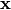is the input to the network. Then the first network operation would be to modify the input via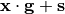using context- dependent gain and shift parameters.

Note

Argument applies only if use_context_mod is True.

• no_last_layer_context_mod (bool) –

If True, context-dependent modulation will not be applied to the output layer.

Note

Argument applies only if use_context_mod is True.

• context_mod_no_weights (bool) –

The weights of the context-mod layers (utils.context_mod_layer.ContextModLayer) are treated independently of the option no_weights. This argument can be used to decide whether the context-mod parameters (gains and shifts) are maintained internally or externally.

Note

Check out argument weights of the forward() method on how to correctly pass weights to the network that are externally maintained.

• context_mod_post_activation (bool) –

Apply context-mod layers after the activation function (activation_fn) in hidden layer rather than before, which is the default behavior.

Note

This option only applies if use_context_mod is True.

Note

This option does not affect argument context_mod_inputs.

Note

Note, there is no non-linearity applied to the output layer, such that this argument has no effect there.

• context_mod_gain_offset (bool) – Activates option apply_gain_offset of class utils.context_mod_layer.ContextModLayer for all context-mod layers that will be instantiated.

• context_mod_gain_softplus (bool) – Activates option apply_gain_softplus of class utils.context_mod_layer.ContextModLayer for all context-mod layers that will be instantiated.

• context_mod_apply_pixel_wise (bool) –

If False, the context-dependent modulation applies a scalar gain and shift to all feature maps in the output of a convolutional layer. When activating this option, the gain and shift will be a per-pixel parameter in all feature maps.

To be more precise, consider the output of a convolutional layer of shape [C,H,W]. If False, there will be C gain and shift parameters for such a layer. Upon activating this option, the number of gain and shift parameters for such a layer will increase to C x H x W.

Initialize the network.

Parameters:
• num_classes – The number of output neurons.

• verbose – Allow printing of general information about the generated network (such as number of weights).

distillation_targets()[source]

Targets to be distilled after training.

See docstring of abstract super method mnets.mnet_interface.MainNetInterface.distillation_targets().

This network does not have any distillation targets.

Returns:

None

forward(x, weights=None, distilled_params=None, condition=None, collect_activations=False)[source]

Compute the outputof this network given the input.

Parameters:
• (....) – See docstring of method mnets.mnet_interface.MainNetInterface.forward(). We provide some more specific information below.

• x

Input image.

Note

We assume the Tensorflow format, where the last entry denotes the number of channels.

• weights (list or dict) –

If a list of parameter tensors is given and context modulation is used (see argument use_context_mod in constructor), then these parameters are interpreted as context- modulation parameters if the length of weights equals 2*len(net.context_mod_layers). Otherwise, the length is expected to be equal to the length of the attribute mnets.mnet_interface.MainNetInterface.param_shapes.

Alternatively, a dictionary can be passed with the possible keywords internal_weights and mod_weights. Each keyword is expected to map onto a list of tensors. The keyword internal_weights refers to all weights of this network except for the weights of the context-modulation layers. The keyword mod_weights, on the other hand, refers specifically to the weights of the context-modulation layers. It is not necessary to specify both keywords.

• condition (int, optional) – Will be passed as argument ckpt_id to the method utils.context_mod_layer.ContextModLayer.forward() for all context-mod layers in this network.

• collect_activations (bool, optional) – If one wants to return the activations in the network. This information can be used for credit assignment later on, in case an alternative to PyTorch its torch.autograd should be used.

Returns:

Tuple containing:

• y: The output of the network.

• layer_activation (optional): The activations of the network. Only returned if collect_activations was set to True. The list will contain the activations of all convolutional and linear layers.

Return type:

(torch.Tensor or tuple)

training: bool

## Interface for Classifiers

A general interface for main networks used in classification tasks. This abstract base class also provides a collection of static helper functions that are useful in classification problems.

class hypnettorch.mnets.classifier_interface.Classifier(num_classes, verbose)[source]

A general interface for classification networks.

Initialize the network.

Parameters:
• num_classes – The number of output neurons.

• verbose – Allow printing of general information about the generated network (such as number of weights).

static accuracy(y, t)[source]

Computing the accuracy between predictions y and targets t. We assume that the argmax of t results in labels as described in the docstring of method “cross_entropy_loss”.

Parameters:
• y – Outputs from the main network.

• t – Targets in form of soft labels or 1-hot encodings.

Returns:

Relative prediction accuracy on the given batch.

static knowledge_distillation_loss(logits, target_logits, target_mapping=None, device=None, T=2.0)[source]

Compute the knowledge distillation loss as proposed by

Hinton et al., “Distilling the Knowledge in a Neural Network”, NIPS Deep Learning and Representation Learning Workshop, 2015. http://arxiv.org/abs/1503.02531

Parameters:
• logits – Unscaled outputs from the main network, i.e., activations of the last hidden layer (unscaled logits).

• target_logits – Target logits, i.e., activations of the last hidden layer (unscaled logits) from the target model. Note, we won’t detach “target_logits” from the graph. Make sure, that you do this before calling this method.

• target_mapping – In continual learning, it might be that the output layer size of a model is growing. Thus, it could be that the model providing the target_logits has a smaller output size than the current model providing the logits. Therefore, one has to provide a mapping, which is a list of indices for logits that state which activations in logits have a corresponding target in target_logits. For instance, if the output layer size just increased by 1 through appending a new output neuron to the current model, the mapping would simply be: target_mapping = list(range(target_logits.shape)).

• device – Current PyTorch device. Only needs to be specified if “target_mapping” is given.

• T – Softmax temperature.

Returns:

Knowledge Distillation (KD) loss.

static logit_cross_entropy_loss(h, t, reduction='mean')[source]

Compute cross-entropy loss for given predictions and targets. Note, we assume that the argmax of the target vectors results in the correct label.

Parameters:
• h – Unscaled outputs from the main network, i.e., activations of the last hidden layer (unscaled logits).

• t – Targets in form os soft labels or 1-hot encodings.

• reduction (str) – The reduction method to be passed to torch.nn.functional.cross_entropy().

Returns:

Cross-entropy loss computed on logits h and labels extracted from target vector t.

property num_classes

Number of output neurons.

Type:

int

static num_hyper_weights(dims)[source]

The number of weights that have to be predicted by a hypernetwork.

Deprecated since version 1.0: Please use method mnets.mnet_interface.MainNetInterface.shapes_to_num_weights() instead.

Parameters:

dims – For instance, the attribute hyper_shapes.

Returns:

(int)

static softmax_and_cross_entropy(h, t, reduction_sum=False)[source]

Compute the cross entropy from logits, allowing smoothed labels (i.e., this function does not require 1-hot targets).

Parameters:
• h – Unscaled outputs from the main network, i.e., activations of the last hidden layer (unscaled logits).

• t – Targets in form os soft labels or 1-hot encodings.

Returns:

Cross-entropy loss computed on logits h and given targets t.

training: bool

## LeNet

This module contains a general classifier template and a LeNet-like network to classify either MNIST or CIFAR-10 images. The network is implemented in a way that it might not have trainable parameters. Instead, the network weights would have to be passed to the forward method. This makes the usage of a hypernetwork (a network that generates the weights of another network) particularly easy.

class hypnettorch.mnets.lenet.LeNet(in_shape=(28, 28, 1), num_classes=10, verbose=True, arch='mnist_large', no_weights=False, init_weights=None, dropout_rate=-1, **kwargs)[source]

Bases: Classifier

The network consists of two convolutional layers followed by two fully- connected layers. See implementation for details.

LeNet was originally introduced in

“Gradient-based learning applied to document recognition”, LeCun et al., 1998.

Though, the implementation provided here has several difference compared to the original LeNet architecture (e.g., the LeNet-5 architecture):

• There is no special connectivity map before the second convolutional layer as described by table 1 in the original paper.

• The dimensions of layers and their activation functions are dfferent.

• The original LeNet-5 has a third fully connected layer with 1x1 kernels.

We mainly use this modified LeNet architecture for MNIST:

• A small architecture with only 21,840 weights.

• A larger architecture with 431,080 weights.

Both of these architectures are typically used for MNIST nowadays.

Note, a variant of this architecture is also used for CIFAR-10, e.g. in

“Bayesian Convolutional Neural Networks with Bernoulli Approximate Variational Inference”, Gal et al., 2015.

and

“Multiplicative Normalizing Flows for Variational Bayesian Neural Networks”, Louizos et al., 2017.

In both these works, the dimensions of the weight parameters are:

main_dims=[[192,3,5,5],,[192,192,5,5],,[1000,4800],
,[10,1000],],


which is an architecture with 5,747,394 weights. Note, the authors used dropout in different configurations, e.g., after each layer, only after the fully-connected layer or no dropout at all.

Parameters:
• in_shape (tuple or list) –

The shape of an input sample.

Note

We assume the Tensorflow format, where the last entry denotes the number of channels.

• num_classes (int) – The number of output neurons.

• verbose (bool) – Allow printing of general information about the generated network (such as number of weights).

• arch (str) –

The architecture to be employed. The following options are available:

• 'mnist_small': A small LeNet with 21,840 weights suitable for MNIST

• 'mnist_large': A larger LeNet with 431,080 weights suitable for MNIST

• 'cifar': A huge LeNet with 5,747,394 weights designed for CIFAR-10.

• no_weights (bool) – If set to True, no trainable parameters will be constructed, i.e., weights are assumed to be produced ad-hoc by a hypernetwork and passed to the forward() method.

• init_weights (optional) – This option is for convinience reasons. The option expects a list of parameter values that are used to initialize the network weights. As such, it provides a convinient way of initializing a network with a weight draw produced by the hypernetwork.

• dropout_rate (float) –

If -1, no dropout will be applied. Otherwise a number between 0 and 1 is expected, denoting the dropout rate.

Dropout will be applied after the convolutional layers (before pooling) and after the first fully-connected layer (after the activation function).

• **kwargs – Keyword arguments regarding context modulation. This class can process the same context-modulation related arguments as class mnets.mlp.MLP. One may additionally specify the argument context_mod_apply_pixel_wise (see class mnets.resnet.ResNet).

Initialize the network.

Parameters:
• num_classes – The number of output neurons.

• verbose – Allow printing of general information about the generated network (such as number of weights).

distillation_targets()[source]

Targets to be distilled after training.

See docstring of abstract super method mnets.mnet_interface.MainNetInterface.distillation_targets().

This network does not have any distillation targets.

Returns:

None

forward(x, weights=None, distilled_params=None, condition=None)[source]

Compute the outputof this network given the input.

Parameters:
• (....) – See docstring of method mnets.mnet_interface.MainNetInterface.forward(). We provide some more specific information below.

• weights (list or dict) – See argument weights of method mnets.mlp.MLP.forward().

• condition (int, optional) – If provided, then this argument will be passed as argument ckpt_id to the method utils.context_mod_layer.ContextModLayer.forward().

Returns:

The output of the network.

Return type:
training: bool

## Multi-Layer Perceptron

Implementation of a fully-connected neural network.

An example usage is as a main model, that doesn’t include any trainable weights. Instead, weights are received as additional inputs. For instance, using an auxilliary network, a so called hypernetwork, see

Ha et al., “HyperNetworks”, arXiv, 2016, https://arxiv.org/abs/1609.09106

class hypnettorch.mnets.mlp.MLP(n_in=1, n_out=1, hidden_layers=(10, 10), activation_fn=ReLU(), use_bias=True, no_weights=False, init_weights=None, dropout_rate=-1, use_spectral_norm=False, use_batch_norm=False, bn_track_stats=True, distill_bn_stats=False, use_context_mod=False, context_mod_inputs=False, no_last_layer_context_mod=False, context_mod_no_weights=False, context_mod_post_activation=False, context_mod_gain_offset=False, context_mod_gain_softplus=False, out_fn=None, verbose=True)[source]

Implementation of a Multi-Layer Perceptron (MLP).

This is a simple fully-connected network, that receives input vectorand outputs a vector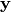of real values.

The output mapping does not include a non-linearity by default, as we wanna map to the whole real line (but see argument out_fn).

Parameters:
• n_in (int) – Number of inputs.

• n_out (int) – Number of outputs.

• hidden_layers (list or tuple) – A list of integers, each number denoting the size of a hidden layer.

• activation_fn – The nonlinearity used in hidden layers. If None, no nonlinearity will be applied.

• use_bias (bool) – Whether layers may have bias terms.

• no_weights (bool) – If set to True, no trainable parameters will be constructed, i.e., weights are assumed to be produced ad-hoc by a hypernetwork and passed to the forward() method.

• init_weights (optional) –

This option is for convinience reasons. The option expects a list of parameter values that are used to initialize the network weights. As such, it provides a convinient way of initializing a network with a weight draw produced by the hypernetwork.

Note, internal weights (see mnets.mnet_interface.MainNetInterface.weights) will be affected by this argument only.

• dropout_rate – If -1, no dropout will be applied. Otherwise a number between 0 and 1 is expected, denoting the dropout rate of hidden layers.

• use_spectral_norm – Use spectral normalization for training.

• use_batch_norm (bool) – Whether batch normalization should be used. Will be applied before the activation function in all hidden layers.

• bn_track_stats (bool) –

If batch normalization is used, then this option determines whether running statistics are tracked in these layers or not (see argument track_running_stats of class utils.batchnorm_layer.BatchNormLayer).

If False, then batch statistics are utilized even during evaluation. If True, then running stats are tracked. When using this network in a continual learning scenario with different tasks then the running statistics are expected to be maintained externally. The argument stats_id of the method utils.batchnorm_layer.BatchNormLayer.forward() can be provided using the argument condition of method forward().

Example

To maintain the running stats, one can simply iterate over all batch norm layers and checkpoint the current running stats (e.g., after learning a task when applying a Continual learning scenario).

for bn_layer in net.batchnorm_layers:
bn_layer.checkpoint_stats()


• distill_bn_stats (bool) –

If True, then the shapes of the batchnorm statistics will be added to the attribute mnets.mnet_interface.MainNetInterface.hyper_shapes_distilled and the current statistics will be returned by the method distillation_targets().

Note, this attribute may only be True if bn_track_stats is True.

• use_context_mod (bool) – Add context-dependent modulation layers utils.context_mod_layer.ContextModLayer after the linear computation of each layer.

• context_mod_inputs (bool) –

Whether context-dependent modulation should also be applied to network intpus directly. I.e., assumeis the input to the network. Then the first network operation would be to modify the input viausing context- dependent gain and shift parameters.

Note

Argument applies only if use_context_mod is True.

• no_last_layer_context_mod (bool) –

If True, context-dependent modulation will not be applied to the output layer.

Note

Argument applies only if use_context_mod is True.

• context_mod_no_weights (bool) –

The weights of the context-mod layers (utils.context_mod_layer.ContextModLayer) are treated independently of the option no_weights. This argument can be used to decide whether the context-mod parameters (gains and shifts) are maintained internally or externally.

Note

Check out argument weights of the forward() method on how to correctly pass weights to the network that are externally maintained.

• context_mod_post_activation (bool) –

Apply context-mod layers after the activation function (activation_fn) in hidden layer rather than before, which is the default behavior.

Note

This option only applies if use_context_mod is True.

Note

This option does not affect argument context_mod_inputs.

Note

This option does not affect argument no_last_layer_context_mod. Hence, if a output-nonlinearity is applied through argument out_fn, then context-modulation would be applied before this non-linearity.

• context_mod_gain_offset (bool) – Activates option apply_gain_offset of class utils.context_mod_layer.ContextModLayer for all context-mod layers that will be instantiated.

• context_mod_gain_softplus (bool) – Activates option apply_gain_softplus of class utils.context_mod_layer.ContextModLayer for all context-mod layers that will be instantiated.

• out_fn (optional) –

If provided, this function will be applied to the output neurons of the network.

Warning

This changes the interpretation of the output of the forward() method.

• verbose (bool) – Whether to print information (e.g., the number of weights) during the construction of the network.

Initializes internal Module state, shared by both nn.Module and ScriptModule.

distillation_targets()[source]

Targets to be distilled after training.

See docstring of abstract super method mnets.mnet_interface.MainNetInterface.distillation_targets().

This method will return the current batch statistics of all batch normalization layers if distill_bn_stats and use_batch_norm was set to True in the constructor.

Returns:

The target tensors corresponding to the shapes specified in attribute hyper_shapes_distilled.

forward(x, weights=None, distilled_params=None, condition=None)[source]

Compute the outputof this network given the input.

Parameters:
• (....) – See docstring of method mnets.mnet_interface.MainNetInterface.forward(). We provide some more specific information below.

• weights (list or dict) –

If a list of parameter tensors is given and context modulation is used (see argument use_context_mod in constructor), then these parameters are interpreted as context- modulation parameters if the length of weights equals 2*len(net.context_mod_layers). Otherwise, the length is expected to be equal to the length of the attribute mnets.mnet_interface.MainNetInterface.param_shapes.

Alternatively, a dictionary can be passed with the possible keywords internal_weights and mod_weights. Each keyword is expected to map onto a list of tensors. The keyword internal_weights refers to all weights of this network except for the weights of the context-modulation layers. The keyword mod_weights, on the other hand, refers specifically to the weights of the context-modulation layers. It is not necessary to specify both keywords.

• distilled_params – Will be passed as running_mean and running_var arguments of method utils.batchnorm_layer.BatchNormLayer.forward() if batch normalization is used.

• condition (int or dict, optional) –

If int is provided, then this argument will be passed as argument stats_id to the method utils.batchnorm_layer.BatchNormLayer.forward() if batch normalization is used.

If a dict is provided instead, the following keywords are allowed:

• bn_stats_id: Will be handled as stats_id of the batchnorm layers as described above.

• cmod_ckpt_id: Will be passed as argument ckpt_id to the method utils.context_mod_layer.ContextModLayer.forward().

Returns:

Tuple containing:

• y: The output of the network.

• h_y (optional): If out_fn was specified in the constructor, then this value will be returned. It is the last hidden activation (before the out_fn has been applied).

Return type:

(tuple)

training: bool
static weight_shapes(n_in=1, n_out=1, hidden_layers=[10, 10], use_bias=True)[source]

Compute the tensor shapes of all parameters in a fully-connected network.

Parameters:
• n_in – Number of inputs.

• n_out – Number of output units.

• hidden_layers – A list of ints, each number denoting the size of a hidden layer.

• use_bias – Whether the FC layers should have biases.

Returns:

A list of list of integers, denoting the shapes of the individual parameter tensors.

## Main-Network Interface

The module mnets.mnet_interface contains an interface for main networks. The interface ensures that we can consistently use these networks without knowing their specific implementation.

class hypnettorch.mnets.mnet_interface.MainNetInterface[source]

Bases: ABC

A general interface for main networks, that can be used stand-alone (i.e., having their own weights) or with no (or only some) internal weights, such that the remaining weights have to be passed through the forward function (e.g., they may be generated through a hypernetwork).

property batchnorm_layers

A list of instances of class utils.batchnorm_layer.BatchNormLayer in case batch normalization is used in this network.

Note

We explicitly do not support the usage of PyTorch its batchnorm layers as class utils.batchnorm_layer.BatchNormLayer represents a hypernet compatible wrapper for them.

Type:

torch.nn.ModuleList

property context_mod_layers

A list of instances of class utils.context_mod_layer.ContextModLayer in case these are used in this network.

Type:

torch.nn.ModuleList

custom_init(normal_init=False, normal_std=0.02, zero_bias=True)[source]

Initialize weight tensors in attribute layer_weight_tensors using Xavier initialization and set bias vectors to 0.

Note

This method will override the default initialization of the network, which is often based on torch.nn.init.kaiming_uniform_() for weight tensors (i.e., attribute layer_weight_tensors) and a uniform init based on fan-in/fan-out for bias vectors (i.e., attribute layer_bias_vectors).

Parameters:
• normal_init (bool) – Use normal initialization rather than Xavier.

• normal_std (float) – The standard deviation when choosing normal_init.

• zero_bias (bool) – Whether bias vectors should be initialized to zero. If False, then bias vectors are left untouched.

abstract distillation_targets()[source]

Targets to be distilled after training.

If hyper_shapes_distilled is not None, then this method can be used to retrieve the targets that should be distilled into an external hypernetwork after training.

The shapes of the returned tensors have to match the shapes specified in hyper_shapes_distilled.

Example

Assume a continual learning scenario with a main network that uses batch normalization (and tracks running statistics). Then this method should be called right after training on a task in order to retrieve the running statistics, such that they can be distilled into a hypernetwork.

Returns:

The target tensors corresponding to the shapes specified in attribute hyper_shapes_distilled.

static flatten_params(params, param_shapes=None, unflatten=False)[source]

Flatten a list of parameter tensors.

This function will take a list of parameter tensors and flatten them into a single vector. This flattening operation can also be undone using the argument unflatten.

Parameters:
• params (list) – A list of tensors. Those tensors will be flattened and concatenated into a tensor. If unflatten=True, then params is expected to be a flattened tensor, which will be split into a list of tensors according to param_shapes.

• param_shapes (list) – List of parameter tensor shapes. Required when unflattening a flattened parameter tensor.

• unflatten (bool) – If True. the flattening operation will be reversed.

Returns:

The flattened tensor. If unflatten=True, a list of tensors will be returned.

Return type:
abstract forward(x, weights=None, distilled_params=None, condition=None)[source]

Compute the outputof this network given the input.

Parameters:
• x – The inputsto the network.

• weights (optional) –

List of weight tensors, that are used as network parameters. If attribute hyper_shapes_learned is not None, then this argument is non-optional and the shapes of the weight tensors have to be as specified by hyper_shapes_learned.

Otherwise, this option might still be set but the weight tensors must follow the shapes specified by attribute param_shapes.

• distilled_params (optional) –

May only be passed if attribute hyper_shapes_distilled is not None.

If not passed but the network relies on those parameters (e.g., batchnorm running statistics), then this method simply chooses the current internal representation of these parameters as returned by distillation_targets().

• condition (optional) –

Sometimes, the network will have to be conditioned on contextual information, which can be passed via this argument and depends on the actual implementation of this interface.

For instance, when using batch normalization in a continual learning scenario, where running statistics have been checkpointed for every task, then this condition might be the actual task ID, that is passed as the argument stats_id of the method utils.batchnorm_layer.BatchNormLayer.forward().

Returns:

The outputof the network.

Create a mask for selecting weights connected solely to certain output units.

This method will return a list of the same length as param_shapes. Entries in this list are either None or masks for the corresponding parameter tensors. For all parameter tensors that are not directly connected to output units, the corresponding entry will be None. If out_inds is None, then all output weights are selected by a masking value 1. Otherwise, only the weights connected to the output units in out_inds are selected, the rest is masked out.

Note

This method only works for networks with a fully-connected output layer (see has_fc_out), that have the attribute mask_fc_out set. Otherwise, the method has to be overwritten by an implementing class.

Parameters:
• out_inds (list, optional) – List of integers. Each entry denotes an output unit.

• device – Pytorch device. If given, the created masks will be moved onto this device.

Returns:

List of masks with the same length as param_shapes. Entries whose corresponding parameter tensors are not connected to the network outputs are None.

Return type:

(list)

property has_bias

Whether layers in this network have bias terms.

Type:

bool

property has_fc_out

Whether the output layer of the network is a fully-connected layer.

Type:

bool

property has_linear_out

Is True if no nonlinearity is applied in the output layer.

Type:

bool

property hyper_shapes_distilled

A list of lists of integers. This attribute is complementary to attribute hyper_shapes_learned, which contains shapes of tensors that are learned through the hypernetwork. In contrast, this attribute should contain the shapes of tensors that are not needed by the main network during training (as it learns or calculates the tensors itself), but should be distilled into a hypernetwork after training in order to avoid increasing memory consumption.

The attribute is None if no tensors have to be distilled into a hypernetwork.

For instance, if batch normalization is used, then the attribute hyper_shapes_learned might contain the batch norm weights whereas the attribute hyper_shapes_distilled contains the running statistics, which are first estimated by the main network during training and later distilled into the hypernetwork.

Type:

list or None

property hyper_shapes_learned

A list of lists of integers. Each list represents the shape of a weight tensor that has to be passed to the forward() method during training. If all weights are maintained internally, then this attribute will be None.

Type:

list

property hyper_shapes_learned_ref

A list of integers. Each entry either represents an index within attribute param_shapes or is set to -1.

Note

The possibility that entries may be -1 should account for unforeseeable flexibility that programmers may need.

Type:

list

property internal_params

A list of all internally maintained parameters of the main network currently in use. If all parameters are assumed to be generated externally, then this attribute will be None.

Simply speaking, the parameters listed here should be passed to the optimizer.

Note

In most cases, the attribute will contain the same set of parameter objects as the method torch.nn.Module.parameters() would return. Though, there might be future use-cases where the programmer wants to hide parameters from the optimizer in a task- or time-dependent manner.

Type:

torch.nn.ParameterList or None

property internal_params_ref

A list of integers. Each entry either represents an index within attribute param_shapes or is set to -1.

Can only be spacified if internal_params is not None.

Note

The possibility that entries may be -1 should account for unforeseeable flexibility that programmers may need.

Type:

list or None

property layer_bias_vectors

Similar to attribute layer_weight_tensors but for the bias vectors in each layer. List should be empty in case has_bias is False.

Note

There might be cases where some weight matrices in attribute layer_weight_tensors have no bias vectors, in which case elements of this list might be None.

Type:

torch.nn.ParameterList

property layer_weight_tensors

These are the actual weight tensors used in layers (e.g., weight matrix in fully-connected layer, kernels in convolutional layer, …).

This attribute is useful when applying a custom initialization to these layers.

Type:

torch.nn.ParameterList

If this attribute is set to True, it is implicitly assumed that if hyper_shapes_learned is not None, the last two entries of hyper_shapes_learned are the weights and biases of the final fully-connected layer.

This attribute is helpful, for instance, in multi-head continual learning settings. In case we regularize task-specific main network weights, it is important to know which weights are specific for an output head (as determined by the weights of the final layer).

Note

Only applies if attribute has_fc_out is True.

Type:

bool

property num_internal_params

The number of internally maintained parameters as prescribed by attribute internal_params.

Type:

int

property num_params

The total number of weights in the parameter tensors described by the attribute param_shapes.

Type:

int

overwrite_internal_params(new_params)[source]

Overwrite the values of all internal parameters.

This will affect all parameters maintained in attribute internal_params.

An example usage of this method is the initialization of a standalone main network with weights that have been previously produced by a hypernetwork.

Parameters:

new_params – A list of parameter values that are used to initialize the network internal parameters is expected.

property param_shapes

A list of lists of integers. Each list represents the shape of a parameter tensor. Note, this attribute is independent of the attribute internal_params, it always comprises the shapes of all parameter tensors as if the network would be stand-alone (i.e., no weights being passed to the forward() method).

Type:

list

property param_shapes_meta

A list of dictionaries. The length of the list is equal to the length of the list param_shapes and each entry of this list provides meta information to the corresponding entry in param_shapes. Each dictionary contains the keys name, index and layer. The key name is a string and refers to the type of weight tensor that the shape corresponds to.

Possible values are:

• 'weight': A weight tensor of a standard layer as those stored in attribute layer_weight_tensors.

• 'bias': A bias vector of a standard layer as those stored in attribute layer_bias_vectors.

• 'bn_scale': The weights for scaling activations in a batchnorm layer utils.batchnorm_layer.BatchNormLayer.

• 'bn_shift': The weights for shifting activations in a batchnorm layer utils.batchnorm_layer.BatchNormLayer.

• 'cm_scale': The weights for scaling activations in a context-mod layer utils.context_mod_layer.ContextModLayer.

• 'cm_shift': The weights for shifting activations in a context-mod layer utils.context_mod_layer.ContextModLayer.

• 'embedding': The parameters represent embeddings.

• None: Not specified!

The key index might refer to the index of the corresponding parameter tensor (if existing) inside the internal_params list. It is -1 if the parameter tensor is not internally maintained.

The key layer is an integer. Shapes with the same layer entry are supposed to reside in the same layer. For instance, a 'weight' and a 'bias' with the same entry for key layer are supposed to be the weight tensor and bias vector in the same layer. The value -1 refers to not specified!

type:

list

static shapes_to_num_weights(dims)[source]

The number of parameters contained in a list of tensors with the given shapes.

Parameters:

dims – List of tensor shapes. For instance, the attribute hyper_shapes_learned.

Returns:

(int)

property weights

Same as internal_params.

Deprecated since version 1.0: Please use attribute internal_params instead.

Type:

torch.nn.ParameterList or None

## ResNet

This module implements the class of Resnet networks described in section 4.2 of the following paper:

“Deep Residual Learning for Image Recognition”, He et al., 2015 https://arxiv.org/abs/1512.03385

class hypnettorch.mnets.resnet.ResNet(in_shape=(32, 32, 3), num_classes=10, use_bias=True, num_feature_maps=(16, 16, 32, 64), verbose=True, n=5, k=1, no_weights=False, init_weights=None, use_batch_norm=True, bn_track_stats=True, distill_bn_stats=False, context_mod_apply_pixel_wise=False, **kwargs)[source]

Bases: Classifier

A resnet with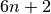layers with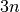residual blocks, consisting of two layers each.

Parameters:
• in_shape (tuple or list) –

The shape of an input sample in format HWC.

Note

We assume the Tensorflow format, where the last entry denotes the number of channels.

• num_classes (int) –

The number of output neurons.

Note

The network outputs logits.

• use_bias (bool) –

Whether layers may have bias terms.

Note

Bias terms are unnecessary in convolutional layers if batch normalization is used. However, this option disables bias terms altogether (including in the final fully-connected layer).

• num_feature_maps (tuple) – A list of 4 integers, each denoting the number of feature maps of convolutional layers in a certain group of the network architecture. The first entry is the number of feature maps of the first convolutional layer, the remaining 3 numbers determine the number of feature maps in the consecutive groups comprising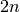convolutional layers each.

• verbose (bool) – Allow printing of general information about the generated network (such as number of weights).

• n (int) – The network will consist oflayers. In the paper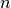has been chosen to be 3, 5, 7, 9 or 18.

• k (int) – The widening factor. Feature maps in the 3 convolutional groups will be multiplied by this number. See argument num_feature_maps. This argument is typical for wide resnets, such as mnets.wide_resnet.WRN. Hence, for k > 1 this network becomes essentially a wide resnet.

• no_weights (bool) –

If set to True, no trainable parameters will be constructed, i.e., weights are assumed to be produced ad-hoc by a hypernetwork and passed to the forward() method.

Note, this also affects the affine parameters of the batchnorm layer. I.e., if set to True, then the argument affine of utils.batchnorm_layer.BatchNormLayer will be set to False and we expect the batchnorm parameters to be passed to the forward().

• init_weights (optional) – This option is for convinience reasons. The option expects a list of parameter values that are used to initialize the network weights. As such, it provides a convinient way of initializing a network with a weight draw produced by the hypernetwork.

• use_batch_norm – Whether batch normalization should used. It will be applied after all convolutional layers (before the activation).

• bn_track_stats

If batch normalization is used, then this option determines whether running statistics are tracked in these layers or not (see argument track_running_stats of class utils.batchnorm_layer.BatchNormLayer).

If False, then batch statistics are utilized even during evaluation. If True, then running stats are tracked. When using this network in a continual learning scenario with different tasks then the running statistics are expected to be maintained externally. The argument stats_id of the method utils.batchnorm_layer.BatchNormLayer.forward() can be provided using the argument condition of method forward().

Example

To maintain the running stats, one can simply iterate over all batch norm layers and checkpoint the current running stats (e.g., after learning a task when applying a Continual Learning scenario).

for bn_layer in net.batchnorm_layers:
bn_layer.checkpoint_stats()


• distill_bn_stats

If True, then the shapes of the batchnorm statistics will be added to the attribute mnets.mnet_interface.MainNetInterface.hyper_shapes_distilled and the current statistics will be returned by the method distillation_targets().

Note, this attribute may only be True if bn_track_stats is True.

• context_mod_apply_pixel_wise (bool) –

By default, the context-dependent modulation applies a scalar gain and shift to all feature maps in the output of a convolutional layer. When activating this option, the gain and shift will be a per-pixel parameter in all feature maps.

To be more precise, consider the output of a convolutional layer of shape [C,H,W]. By default, there will be C gain and shift parameters for such a layer. Upon activating this option, the number of gain and shift parameters for such a layer will increase to C x H x W.

• **kwargs

Keyword arguments regarding context modulation. This class can process the same context-modulation related arguments as class mnets.mlp.MLP. Additionally, one may specify the argument context_mod_apply_pixel_wise.

Some additional remarks regarding the handling of keyword arguments:

• use_context_mod: Context-modulation will be applied after the linear computation of each layer (i.e. all hidden layers (conv layers) as well as the final FC output layer).

Similar to Spatial Batch-Normalization, there will be a scalar shift and gain applied per feature map for all convolutional layers (except if context_mod_apply_pixel_wise is set).

• context_mod_inputs: The input is treated like the output of a convolutional layer when applying context-dependent modulation.

Initialize the network.

Parameters:
• num_classes – The number of output neurons.

• verbose – Allow printing of general information about the generated network (such as number of weights).

distillation_targets()[source]

Targets to be distilled after training.

See docstring of abstract super method mnets.mnet_interface.MainNetInterface.distillation_targets().

This method will return the current batch statistics of all batch normalization layers if distill_bn_stats and use_batch_norm were set to True in the constructor.

Returns:

The target tensors corresponding to the shapes specified in attribute hyper_shapes_distilled.

forward(x, weights=None, distilled_params=None, condition=None)[source]

Compute the outputof this network given the input.

Parameters:
• (....) – See docstring of method mnets.mnet_interface.MainNetInterface.forward(). We provide some more specific information below.

• x (torch.Tensor) –

Batch of flattened input images.

Note

We assume the Tensorflow format, where the last entry denotes the number of channels.

• weights (list or dict) –

If a list of parameter tensors is given and context modulation is used (see argument use_context_mod in constructor), then these parameters are interpreted as context- modulation parameters if the length of weights equals 2*len(net.context_mod_layers). Otherwise, the length is expected to be equal to the length of the attribute mnets.mnet_interface.MainNetInterface.param_shapes.

Alternatively, a dictionary can be passed with the possible keywords internal_weights and mod_weights. Each keyword is expected to map onto a list of tensors. The keyword internal_weights refers to all weights of this network except for the weights of the context-modulation layers. The keyword mod_weights, on the other hand, refers specifically to the weights of the context-modulation layers. It is not necessary to specify both keywords.

• distilled_params – Will be passed as running_mean and running_var arguments of method utils.batchnorm_layer.BatchNormLayer.forward() if batch normalization is used.

• condition (optional, int or dict) –

If int is provided, then this argument will be passed as argument stats_id to the method utils.batchnorm_layer.BatchNormLayer.forward() if batch normalization is used.

If a dict is provided instead, the following keywords are allowed:

• bn_stats_id: Will be handled as stats_id of the batchnorm layers as described above.

• cmod_ckpt_id: Will be passed as argument ckpt_id to the method utils.context_mod_layer.ContextModLayer.forward().

Returns:

The output of the network.

Return type:
training: bool

## ResNet for ImageNet

This module implements the class of Resnet networks described Table 1 of the following paper:

“Deep Residual Learning for Image Recognition”, He et al., 2015 https://arxiv.org/abs/1512.03385

Those networks are designed for inputs of size 224 x 224. In contrast, the Resnet family implemented by class mnets.resnet.ResNet is primarily designed for CIFAR like inputs of size 32 x 32.

class hypnettorch.mnets.resnet_imgnet.ResNetIN(in_shape=(224, 224, 3), num_classes=1000, use_bias=True, use_fc_bias=None, num_feature_maps=(64, 64, 128, 256, 512), blocks_per_group=(2, 2, 2, 2), projection_shortcut=False, bottleneck_blocks=False, cutout_mod=False, no_weights=False, use_batch_norm=True, bn_track_stats=True, distill_bn_stats=False, chw_input_format=False, verbose=True, **kwargs)[source]

Bases: Classifier

Hypernet-compatible Resnets for ImageNet.

The architecture of those Resnets is summarized in Table 4 of He et al.. They consist of 5 groups of convolutional layers, where the first group only has 1 convolutional layer followed by a max-pooling operation. The other 4 groups consist of blocks (see blocks_per_group) of either 2 or 3 (see bottleneck_blocks) convolutional layers per block. The network then computes its output via a final average pooling operation and a fully- connected layer.

The number of layer per network is therewith 1 + sum(blocks_per_group) * 2 + 1, i.e., initial conv layer, num. conv layers in all blocks (assuming bottleneck_blocks=False) and the final fully-connected layer. If projection_shortcut=True, additional 1x1 conv layers are added for shortcuts where the feature maps tensor shape changes.

Here are a few implementation details worth noting:
• If use_batch_norm=True, it would be redundant to add convolutional layers to the conv layers, therefore one should set use_bias=False, use_fc_bias=True. Skip connections never use biases.

• Online implementations vary in their use of projection or identity shortcuts. We offer both possibilities (projection_shortcut). If projection_shortcut is used, then a batchnorm layer is added after each projection.

Here are parameter configurations that can be used to obtain well-known Resnets (all configurations should use use_bias=False, use_fc_bias=True):

• Resnet-18: blocks_per_group=(2,2,2,2), bottleneck_blocks=False

• Resnet-34: blocks_per_group=(3,4,6,3), bottleneck_blocks=False

• Resnet-50: blocks_per_group=(3,4,6,3), bottleneck_blocks=True

• Resnet-101: blocks_per_group=(3,4,23,3), bottleneck_blocks=True

• Resnet-152: blocks_per_group=(3,4,36,3), bottleneck_blocks=True

Parameters:
• (....) – See arguments of class:mnets.wrn.WRN.

• num_feature_maps (tuple) –

A list of 5 integers, each denoting the number of feature maps in a group of convolutional layers.

Note

If bottleneck_blocks=True, then the last 1x1 conv layer in each block has 4 times as many feature maps as specified by this argument.

• blocks_per_group (tuple) – A list of 4 integers, each denoting the number of convolutional blocks for the groups of convolutional layers.

• projection_shortcut (bool) – If True, skip connections that otherwise would require zero-padding or subsampling will be realized via 1x1 conv layers followed by batchnorm. All other skip connections will be realized via identity mappings.

• bottleneck_blocks (bool) – Whether normal blocks or bottleneck blocks should be used (cf. Fig. 5 in He et al.)

• cutout_mod (bool) –

Sometimes, networks from this family are used for smaller (CIFAR-like) images. In this case, one has to either upscale the images or adapt the architecture slightly (otherwise, small images are too agressively downscaled at the very beginning).

When activating this option, the first conv layer is modified as described here, i.e., it uses a kernel size of 3 with stride 1 and the max-pooling layer is omitted.

Note, in order to recover the same architecture as in the link above one has to additionally set: use_bias=False, use_fc_bias=True, projection_shortcut=True.

Initialize the network.

Parameters:
• num_classes – The number of output neurons.

• verbose – Allow printing of general information about the generated network (such as number of weights).

distillation_targets()[source]

Targets to be distilled after training.

See docstring of abstract super method mnets.mnet_interface.MainNetInterface.distillation_targets().

This method will return the current batch statistics of all batch normalization layers if distill_bn_stats and use_batch_norm were set to True in the constructor.

Returns:

The target tensors corresponding to the shapes specified in attribute hyper_shapes_distilled.

forward(x, weights=None, distilled_params=None, condition=None)[source]

Compute the outputof this network given the input.

Parameters:
• (....) – See docstring of method mnets.resnet.ResNet.forward(). We provide some more specific information below.

• x (torch.Tensor) – Based on the constructor argument chw_input_format, either a flattened image batch with encoding HWC or an unflattened image batch with encoding CHW is expected.

Returns:

The output of the network.

Return type:

Create a mask for selecting weights connected solely to certain output units.

See docstring of overwritten super method mnets.mnet_interface.MainNetInterface.get_output_weight_mask().

property has_bias

Getter for read-only attribute has_bias.

training: bool

## SimpleRNN

Implementation of a simple recurrent neural network that has stacked vanilla RNN or LSTM layers that are optionally enclosed by fully-connected layers.

An example usage is as a main model, where the main weights are initialized and protected by a method such as EWC, and the context-modulation patterns of the neurons are produced by an external hypernetwork.

class hypnettorch.mnets.simple_rnn.SimpleRNN(n_in=1, rnn_layers=(10,), fc_layers_pre=(), fc_layers=(1,), activation=Tanh(), use_lstm=False, use_bias=True, no_weights=False, init_weights=None, kaiming_rnn_init=False, context_mod_last_step=False, context_mod_num_ts=-1, context_mod_separate_layers_per_ts=False, verbose=True, **kwargs)[source]

Implementation of a simple RNN.

This is a simple recurrent network, that receives input vectorand outputs a vectorof real values.

Note

The output is non-linear if the last layer is recurrent! Otherwise, logits are returned (cmp. attribute mnets.mnet_interface.MainNetInterface.has_fc_out).

Parameters:
• n_in (int) – Number of inputs.

• rnn_layers (list or tuple) –

List of integers. Each entry denotes the size of a recurrent layer. Recurrent layers will simply be stacked as layers of this network.

If fc_layers_pre is empty, then the recurrent layers are the initial layers. If fc_layers is empty, then the last entry of this list will denote the output size.

Note

This list may never be empty.

• fc_layers_pre (list or tuple) –

List of integers. Before the recurrent layers a set of fully-connected layers may be added. This might be specially useful when constructing recurrent autoencoders. The entries of this list will denote the sizes of those layers.

If fc_layers_pre is not empty, its first entry will denote the input size of this network.

• fc_layers (list or tuple) –

List of integers. After the recurrent layers, a set of fully-connected layers is added. The entries of this list will denote the sizes of those layers.

If fc_layers is not empty, its last entry will denote the output size of this network.

• activation – The nonlinearity used in hidden layers.

• use_lstm (bool) – If set to True, the recurrent layers will be LSTM layers.

• use_bias (bool) – Whether layers may have bias terms.

• no_weights (bool) – If set to True, no trainable parameters will be constructed, i.e., weights are assumed to be produced ad-hoc by a hypernetwork and passed to the forward() method.

• init_weights (list, optional) –

This option is for convinience reasons. The option expects a list of parameter values that are used to initialize the network weights. As such, it provides a convinient way of initializing a network with a weight draw produced by the hypernetwork.

Note, internal weights (see mnets.mnet_interface.MainNetInterface.weights) will be affected by this argument only.

• kaiming_rnn_init (bool) –

By default, PyTorch initializes its recurrent layers uniformly with an interval defined by the square-root of the inverse of the layer size.

If this option is enabled, then the recurrent layers will be initialized using the kaiming init as implemented by the function utils.torch_utils.init_params().

• context_mod_last_step (bool) –

Whether context modulation is applied at the last time step os a recurrent layer only. If False, context modulation is applied at every time step.

Note

This option only applies if use_context_mod is True.

• context_mod_num_ts (int, optional) –

The maximum number of timesteps. If specified, context-modulation with a different set of weights is applied at every timestep. If context_mod_separate_layers_per_ts is True, then a separate context-mod layer per timestep will be created. Otherwise, a single context-mod layer is created, but the expected parameter shapes for this layer are [context_mod_num_ts, *context_mod_shape].

Note

This option only applies if use_context_mod is True.

• context_mod_separate_layers_per_ts (bool) –

If specified, a separate context-mod layer per timestep is created (required if context_mod_no_weights is False).

Note

Only applies if context_mod_num_ts is specified.

• verbose (bool) – Whether to print information (e.g., the number of weights) during the construction of the network.

• **kwargs – Keyword arguments regarding context modulation. This class can process the same context-modulation related arguments as class mnets.mlp.MLP (plus the additional ones noted above).

Initializes internal Module state, shared by both nn.Module and ScriptModule.

basic_rnn_step(d, t, x_t, h_t, int_weights, cm_weights, ckpt_id, is_last_step)[source]

Perform vanilla rnn pass from inputs to hidden units.

Apply context modulation if necessary (i.e. if cm_weights is not None).

This function implements a step of an Elman RNN.

Note

We made the following design choice regarding context-modulation. In contrast to the LSTM, the Elman network layer consists of “two steps”, updating the hidden state and computing an output based on this hidden state. To be fair, context-mod should influence both these “layers”. Therefore, we apply context-mod twice, but using the same weights. This of course assumes that the hidden state and output vector have the same dimensionality.

Parameters:
• d (int) – Index of the layer.

• t (int) – Current timestep.

• x_t – Tensor of size [batch_size, n_hidden_prev] with inputs.

• h_t (tuple) –

Tuple of length 2, containing two tensors of size [batch_size, n_hidden] with previous hidden states h and and previous outputs y.

Note

The previous outputs y are ignored by this method, since they are not required in an Elman RNN step.

• int_weights – See docstring of method compute_hidden_states().

• cm_weights (list) – The weights of the context-mod layer, if context- mod should be applied.

• ckpt_id – See docstring of method compute_hidden_states().

• is_last_step (bool) – Whether the current time step is the last one.

Returns:

Tuple containing:

• h_t (torch.Tensor): The tensor h_t of size [batch_size, n_hidden] with the new hidden state.

• y_t (torch.Tensor): The tensor y_t of size [batch_size, n_hidden] with the new cell state.

Return type:

(tuple)

property bptt_depth

The truncation depth for backprop through time.

If -1, backprop through time (BPTT) will unroll all timesteps present in the input. Otherwise, the forward pass will detach the RNN hidden states smaller or equal than num_timesteps - bptt_depth timesteps, resulting in truncated BPTT (T-BPTT).

Type:

int

compute_basic_rnn_output(h_t, int_weights, use_cm, cm_weights, cm_idx, ckpt_id, is_last_step)[source]

Compute the output of a vanilla RNN given the hidden state.

Parameters:
• (...) – See docstring of method basic_rnn_step().

• use_cm (boolean) – Whether context modulation is being used.

• cm_idx (int) – Index of the context-mod layer.

Returns:

The output.

Return type:

(torch.tensor)

compute_fc_outputs(h, fc_w_weights, fc_b_weights, num_fc_cm_layers, cm_fc_layer_weights, cm_offset, cmod_cond, is_post_fc, ret_hidden)[source]

Compute the forward pass through the fully-connected layers.

This method also appends activations to ret_hidden.

Parameters:
• h (torch.Tensor) – The input from the previous layer.

• fc_w_weights (list) – The weights for the fc layers.

• fc_b_weights (list) – The biases for the fc layers.

• num_fc_cm_layers (int) – The number of context-modulation layers associated with this set of fully-connected layers.

• cm_fc_layer_weights (list) – The context-modulation weights associated with the current layers.

• cm_offset (int) – The index to access the correct context-mod layers.

• cmod_cond (bool) – Some condition to perform context modulation.

• is_post_fc (bool) – layers of the network. In this case, there will be no activation applied to the last layer outputs.

• ret_hidden (list or None) – The list where to append the hidden recurrent activations.

Returns:

Tuple containing:

• ret_hidden: The hidden recurrent activations.

• h: Transformed activation h.

Return type:

(Tuple)

compute_hidden_states(x, layer_ind, int_weights, cm_weights, ckpt_id, h_0=None, c_0=None)[source]

Compute the hidden states for the recurrent layer layer_ind from a sequence of inputs.

If so specified, context modulation is applied before or after the nonlinearities.

Parameters:
• x – The inputsto the layer.has shape [sequence_len, batch_size, n_hidden_prev].

• layer_ind (int) – Index of the layer.

• int_weights – Internal weights associated with this recurrent layer.

• cm_weights – Context modulation weights.

• ckpt_id – Will be passed as option ckpt_id to method utils.context_mod_layer.ContextModLayer.forward() if context-mod layers are used.

• h_0 (torch.Tensor, optional) – The initial state for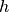.

• c_0 (torch.Tensor, optional) – The initial state for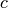. Note that for LSTMs, if the initial state is to be defined, this variable is necessary also, not only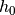, whereas for vanilla RNNs it is enough to provideas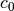represents the output of the layer and it can be easily computed from h_0.

Returns:

Tuple containing:

• outputs (torch.Tensor): The sequence of visible hidden states given the input. It has shape [sequence_len, batch_size, n_hidden].

• hiddens (torch.Tensor): The sequence of hidden states given the input. For LSTMs, this corresponds to. It has shape [sequence_len, batch_size, n_hidden].

Return type:

(tuple)

distillation_targets()[source]

Targets to be distilled after training.

See docstring of abstract super method mnets.mnet_interface.MainNetInterface.distillation_targets().

This network does not have any distillation targets.

Returns:

None

forward(x, weights=None, distilled_params=None, condition=None, return_hidden=False, return_hidden_int=False)[source]

Compute the outputof this network given the input.

Parameters:
• (....) – See docstring of method mnets.mnet_interface.MainNetInterface.forward(). We provide some more specific information below.

• weights (list or dict) – See argument weights of method mnets.mlp.MLP.forward().

• condition (optional, int) – If provided, then this argument will be passed as argument ckpt_id to the method utils.context_mod_layer.ContextModLayer.forward().

• return_hidden (bool, optional) –

If True, all hidden activations of fully-connected and recurrent layers (where we defined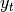as hidden state of vannila RNN layers as these are the layer output passed to the next layer) are returned.

Specifically, hidden activations are the outputs of each hidden layer that are passed to the next layer.

• return_hidden_int (bool, optional) – If True, in addition to hidden, an additional variable hidden_int is returned containing the internal hidden states of recurrent layers (i.e., the cell states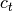for LSTMs and the actual hidden state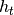for Elman layers) are returned. Since fully- connected layers have no such internal hidden activations, the corresponding entry in hidden_int will be None.

Returns:

Where the tuple is containing:

• output (torch.Tensor): The output of the network.

• hidden (list): If return_hidden is True, then the hidden activities of the layers are returned, which have the shape (seq_length, batch_size, n_hidden).

• hidden_int (list): If return_hidden_int is True, then in addition to hidden a tensor hidden_int per recurrent layer is returned containing internal hidden states. The list will contain a None entry for each fully-connected layer to ensure same length as hidden.

Return type:
get_cm_inds()[source]

Get the indices of mnets.mnet_interface.MainNetInterface.param_shapes that are associated with context-modulation.

Returns:

List of integers representing indices of mnets.mnet_interface.MainNetInterface.param_shapes.

Return type:

(list)

get_cm_weights()[source]

Get internal maintained weights that are associated with context- modulation.

Returns:

List of weights from mnets.mnet_interface.MainNetInterface.internal_params that are belonging to context-mod layers.

Return type:

(list)

get_non_cm_weights()[source]

Get internal weights that are not associated with context-modulation.

Returns:

List of weights from mnets.mnet_interface.MainNetInterface.internal_params that are not belonging to context-mod layers.

Return type:

(list)

Get masks to select output weights.

See docstring of overwritten super method mnets.mnet_interface.MainNetInterface.get_output_weight_mask().

init_hh_weights_orthogonal()[source]

Initialize hidden-to-hidden weights orthogonally.

This method will overwrite the hidden-to-hidden weights of recurrent layers.

lstm_rnn_step(d, t, x_t, h_t, int_weights, cm_weights, ckpt_id, is_last_step)[source]

Perform an LSTM pass from inputs to hidden units.

Apply masks to the temporal sequence for computing the loss. Obtained from:

and:

Parameters:
Returns:

Tuple containing:

• h_t (torch.Tensor): The tensor h_t of size [batch_size, n_hidden] with the new hidden state.

• c_t (torch.Tensor): The tensor c_t of size [batch_size, n_hidden] with the new cell state.

Return type:

(tuple)

property num_rec_layers

Number of recurrent layers in this network (i.e., length of constructor argument rnn_layers).

Type:

int

split_cm_weights(cm_weights, condition, num_ts=0)[source]

Split context-mod weights per context-mod layer.

Parameters:
• cm_weights (torch.Tensor) – All context modulation weights.

• condition (optional, int) – If provided, then this argument will be passed as argument ckpt_id to the method utils.context_mod_layer.ContextModLayer.forward().

• num_ts (int) – The length of the sequences.

Returns:

Where the tuple contains:

• cm_inputs_weights: The cm input weights.

• cm_fc_pre_layer_weights: The cm pre-recurrent weights.

• cm_rec_layer_weights: The cm recurrent weights.

• cm_fc_layer_weights: The cm post-recurrent weights.

• n_cm_rec: The number of recurrent cm layers.

• cmod_cond: The context-mod condition.

Return type:

(Tuple)

split_internal_weights(int_weights)[source]

Split internal weights per layer.

Parameters:

int_weights (torch.Tensor) – All internal weights.

Returns:

Where the tuple contains:

• fc_pre_w_weights: The pre-recurrent w weights.

• fc_pre_b_weights: The pre-recurrent b weights.

• rec_weights: The recurrent weights.

• fc_w_weights:The post-recurrent w weights.

• fc_b_weights: The post-recurrent b weights.

Return type:

(Tuple)

split_weights(weights)[source]

Split weights into internal and context-mod weights.

Extract which weights should be used, I.e., are we using internally maintained weights or externally given ones or are we even mixing between these groups.

Parameters:

weights (torch.Tensor) – All weights.

Returns:

Where the tuple contains:

• int_weights: The internal weights.

• cm_weights: The context-mod weights.

Return type:

(Tuple)

training: bool
property use_lstm

See constructor argument use_lstm.

Type:

bool

## Wide-ResNet

The module mnets.wide_resnet implements the class of Wide Residual Networks as described in:

Zagoruyko et al., “Wide Residual Networks”, 2017.

class hypnettorch.mnets.wide_resnet.WRN(in_shape=(32, 32, 3), num_classes=10, n=4, k=10, num_feature_maps=(16, 16, 32, 64), use_bias=True, use_fc_bias=None, no_weights=False, use_batch_norm=True, bn_track_stats=True, distill_bn_stats=False, dropout_rate=-1, chw_input_format=False, verbose=True, **kwargs)[source]

Bases: Classifier

Hypernet-compatible Wide Residual Network (WRN).

In the documentation of this class, we follow the notation of the original paper:

•- deepening factor (number of convolutional layers per residual block). In our case,is always going to be 2, as this was the configuration found to work best by the authors.

•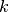- widening factor (multiplicative factor for the number of features in a convolutional layer, see argument k).

•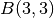- the block structure. The numbers denote the size of the quadratic kernels used in each convolutional layer from a block. Note, the authors found thatworks best, which is why we use this configuration.

•- total number of convolutional layers. Note, here we deviate from the original notation (where this quantity is called). Though, we want our notation to stay consistent with the one used in class mnets.resnet.ResNet.

•- number of residual blocks in a group. Note, a resnet consists of 3 groups of residual blocks. See also argument n of class mnets.resnet.ResNet.

Given this notation, the original paper denotes a WRN architecture via the following notation: WRN-d-k-B(3,3). Note,contains the total number of convolutional layers (including the input layer and all residual connections that are realized via 1x1 convolutions), but it does not contain the final fully-connected layer. The total depth of the network (assuming residual connection do not add to this depth) remainsas for mnets.resnet.ResNet.

Notable implementation differences to mnets.resnet.ResNet (some differences might vanish in the future, this list was updated on 05/06/2020):

• Within a block, convolutional layers are preceeded by a batchnorm layer and the application of the nonlinearity. This changes the structure within a block and therefore, residual connections interface with the network at different locations than in class mnets.resnet.ResNet.

• Dropout can be used. It will act right after the first convolutional layer of each block.

• If the number of feature maps differs along a skip connection or a downsampling has been applied, 1x1 convolutions rather than padding and manual downsampling is used.

Parameters:
• in_shape (tuple or list) –

The shape of an input sample in format HWC.

Note

We assume the Tensorflow format, where the last entry denotes the number of channels. Also, see argument chw_input_format.

• num_classes (int) –

The number of output neurons.

Note

The network outputs logits.

• n (int) – The number of residual blocks per group.

• k (int) – The widening factor. Feature maps in the 3 convolutional groups will be multiplied by this number. See argument num_feature_maps.

• num_feature_maps (tuple) –

A list of 4 integers, each denoting the number of feature maps of convolutional layers in a certain group of the network architecture. The first entry is the number of feature maps of the first convolutional layer, the remaining 3 numbers determine the number of feature maps in the consecutive groups comprisingconvolutional layers each.

Note

The last 3 entries of this list are multiplied by the factor k. use_bias (bool): Whether layers may have bias terms.

• use_bias (bool) –

Whether layers may have bias terms.

Note

Bias terms are unnecessary in convolutional layers if batch normalization is used. However, this option disables bias terms altogether (including in the final fully-connected layer). See option use_fc_bias.

• use_fc_bias (optional, bool) – If None, the value will be linked to use_bias. Otherwise, this option can alter the usage of bias terms in the final layer compared to the remaining (convolutional) layers in the network.

• no_weights (bool) –

If set to True, no trainable parameters will be constructed, i.e., weights are assumed to be produced ad-hoc by a hypernetwork and passed to the forward() method.

Note, this also affects the affine parameters of the batchnorm layer. I.e., if set to True, then the argument affine of utils.batchnorm_layer.BatchNormLayer will be set to False and we expect the batchnorm parameters to be passed to the forward().

• use_batch_norm (bool) – Whether batch normalization should used. There will be a batchnorm layer after each convolutional layyer (excluding possible 1x1 conv layers in the skip connections). However, the logical order is as follows: batchnorm layer -> ReLU -> convolutional layer. Hence, a residual block (containing multiple of these logical units) starts before a batchnorm layer and ends after a convolutional layer.

• bn_track_stats (bool) – See argument bn_track_stats of class mnets.resnet.ResNet.

• distill_bn_stats (bool) – See argument bn_track_stats of class mnets.resnet.ResNet.

• dropout_rate (float) –

If -1, no dropout will be applied. Otherwise a number between 0 and 1 is expected, denoting the dropout rate.

Dropout will be applied after the first convolutional layers (and before the second batchnorm layer) in each residual block.

• chw_input_format (bool) – Due to legacy reasons, the network expects by default flattened images as input that were encoded in the HWC format. When enabling this option, the network expects unflattened images in the CHW format (as typical for PyTorch).

• verbose (bool) – Allow printing of general information about the generated network (such as number of weights).

• **kwargs – Keyword arguments regarding context modulation. This class can process the same context-modulation related arguments as class mnets.mlp.MLP. One may additionally specify the argument context_mod_apply_pixel_wise (see class mnets.resnet.ResNet).

Initialize the network.

Parameters:
• num_classes – The number of output neurons.

• verbose – Allow printing of general information about the generated network (such as number of weights).

distillation_targets()[source]

Targets to be distilled after training.

See docstring of abstract super method mnets.mnet_interface.MainNetInterface.distillation_targets().

This method will return the current batch statistics of all batch normalization layers if distill_bn_stats and use_batch_norm were set to True in the constructor.

Returns:

The target tensors corresponding to the shapes specified in attribute hyper_shapes_distilled.

forward(x, weights=None, distilled_params=None, condition=None)[source]

Compute the outputof this network given the input.

Parameters:
• (....) – See docstring of method mnets.resnet.ResNet.forward(). We provide some more specific information below.

• x (torch.Tensor) – Based on the constructor argument chw_input_format, either a flattened image batch with encoding HWC or an unflattened image batch with encoding CHW is expected.

Returns:

The output of the network.

Return type:

Create a mask for selecting weights connected solely to certain output units.

See docstring of overwritten super method mnets.mnet_interface.MainNetInterface.get_output_weight_mask().

property has_bias

Getter for read-only attribute has_bias.

training: bool

## The Convnet used by Zenke et al. for CIFAR-10/100

The module mnets/zenkenet contains a reimplementation of the network that was used in

“Continual Learning Through Synaptic Intelligence”, Zenke et al., 2017. https://arxiv.org/abs/1703.04200

class hypnettorch.mnets.zenkenet.ZenkeNet(in_shape=(32, 32, 3), num_classes=10, verbose=True, arch='cifar', no_weights=False, init_weights=None, dropout_rate=0.25)[source]

Bases: Classifier

The network consists of four convolutional layers followed by two fully- connected layers. See implementation for details.

ZenkeNet is a network introduced in

“Continual Learning Through Synaptic Intelligence”, Zenke et al., 2017.

See Appendix for details.

We use the same network for a fair comparison to the results reported in the paper.

Parameters:
• in_shape (tuple or list) –

The shape of an input sample.

Note

We assume the Tensorflow format, where the last entry denotes the number of channels.

• num_classes (int) – The number of output neurons. The chosen architecture (see arch) will be adopted accordingly.

• verbose (bool) – Allow printing of general information about the generated network (such as number of weights).

• arch (str) –

The architecture to be employed. The following options are available.

• cifar: The convolutional network used by Zenke et al. for their proposed split CIFAR-10/100 experiment.

• no_weights (bool) – If set to True, no trainable parameters will be constructed, i.e., weights are assumed to be produced ad-hoc by a hypernetwork and passed to the forward() method.

• init_weights (optional) – This option is for convinience reasons. The option expects a list of parameter values that are used to initialize the network weights. As such, it provides a convinient way of initializing a network with a weight draw produced by the hypernetwork.

• dropout_rate (float) –

If -1, no dropout will be applied. Otherwise a number between 0 and 1 is expected, denoting the dropout rate.

Dropout will be applied after the convolutional layers (before pooling) and after the first fully-connected layer (after the activation function).

Note

For the FC layer, the dropout rate is doubled.

Initialize the network.

Parameters:
• num_classes – The number of output neurons.

• verbose – Allow printing of general information about the generated network (such as number of weights).

distillation_targets()[source]

Targets to be distilled after training.

See docstring of abstract super method mnets.mnet_interface.MainNetInterface.distillation_targets().

This network does not have any distillation targets.

Returns:

None

forward(x, weights=None, distilled_params=None, condition=None)[source]

Compute the outputof this network given the input.

Parameters:
• (....) – See docstring of method mnets.mnet_interface.MainNetInterface.forward()`. We provide some more specific information below.

• x

Input image.

Note

We assume the Tensorflow format, where the last entry denotes the number of channels.

Returns:

The output of the network.

Return type:

y

training: bool